Ex 2.1

Chapter 2 Class 12 Inverse Trigonometric Functions
Serial order wise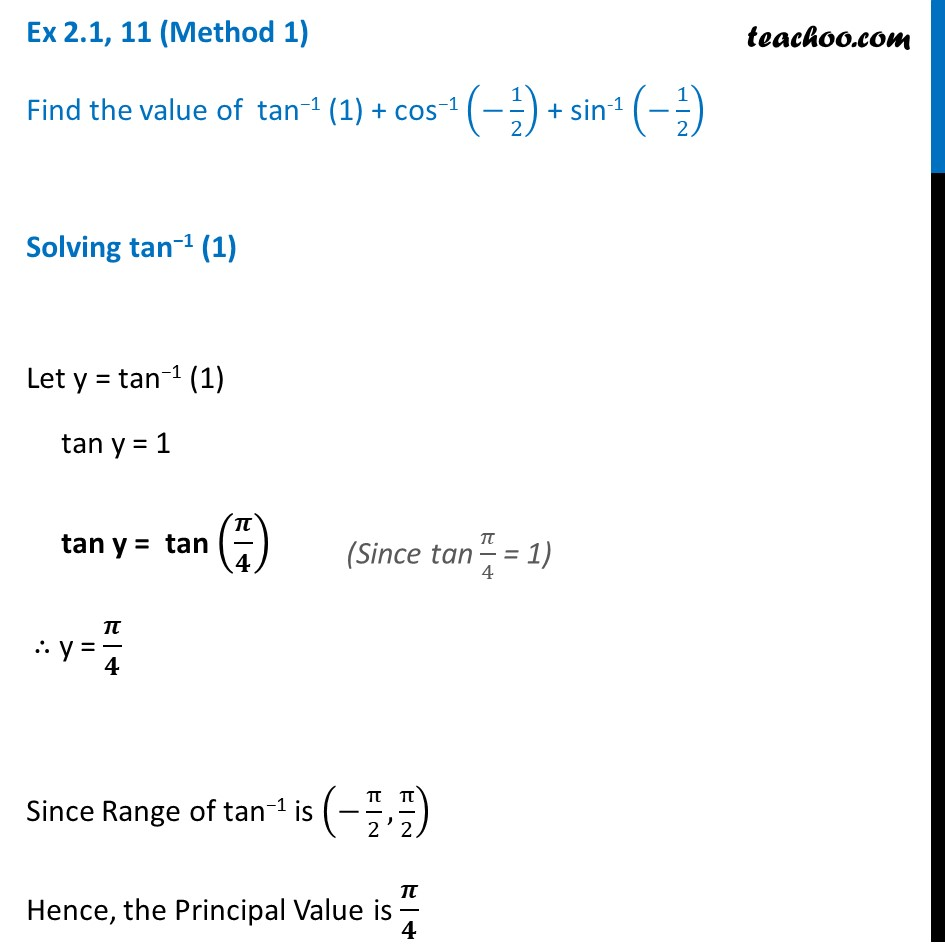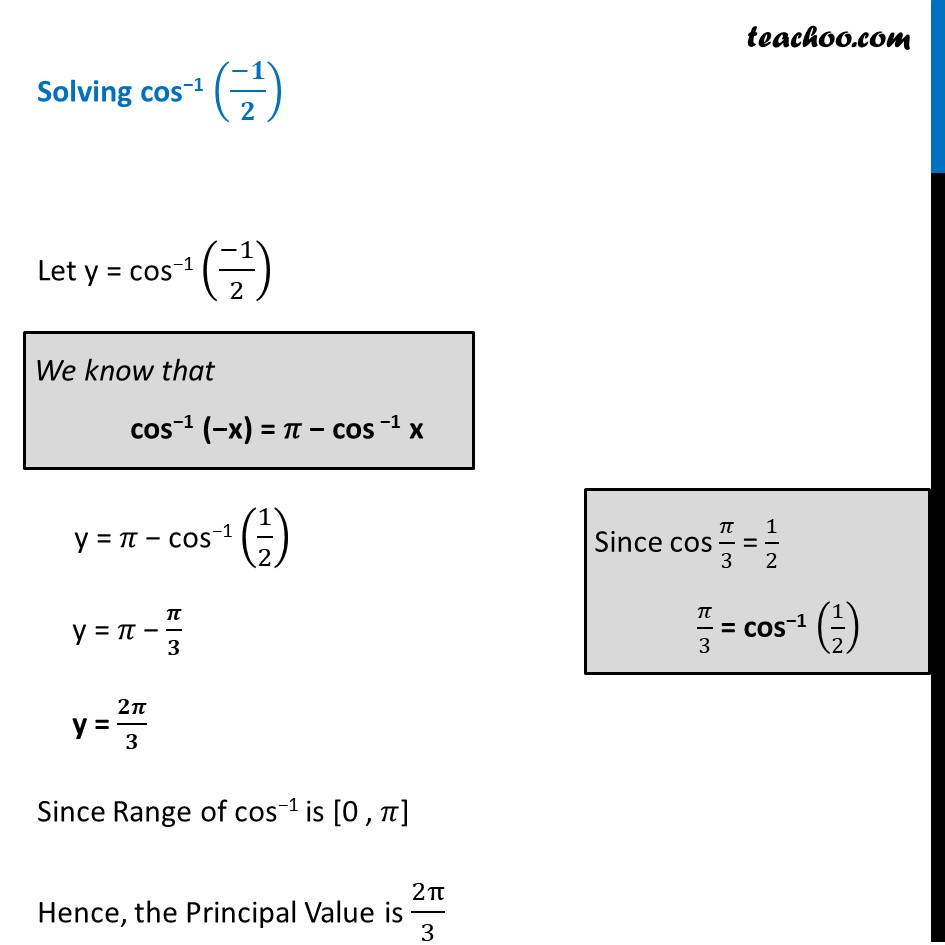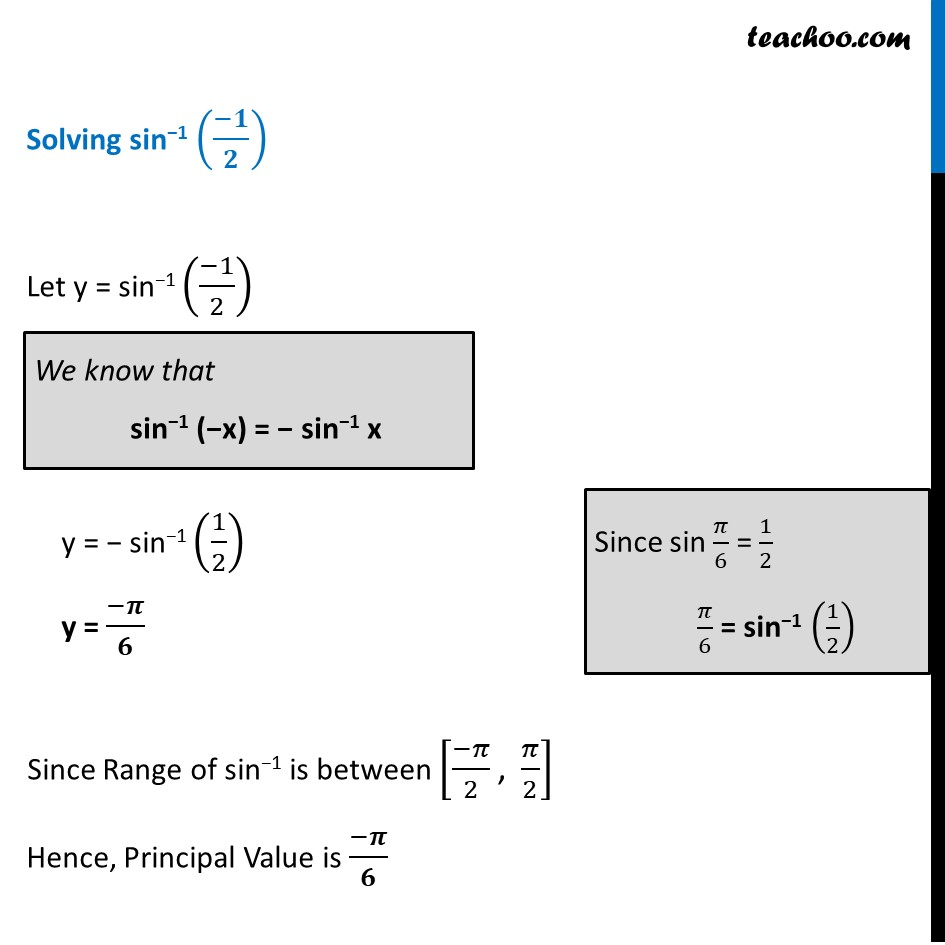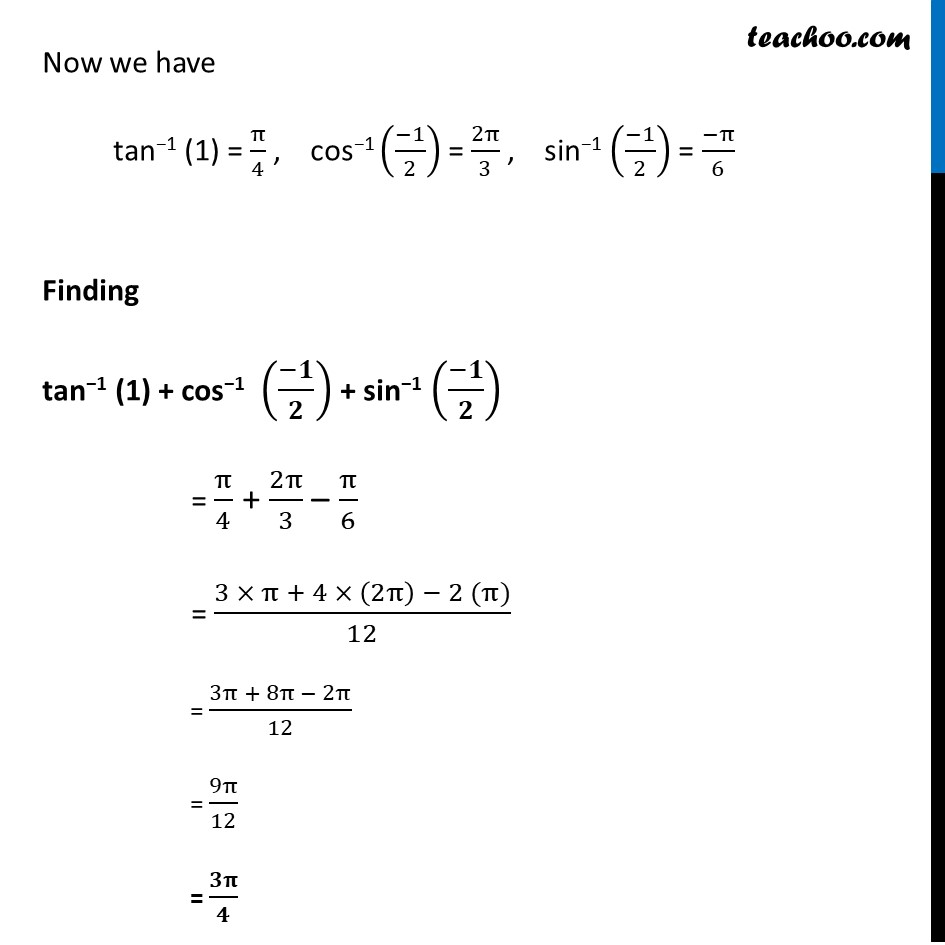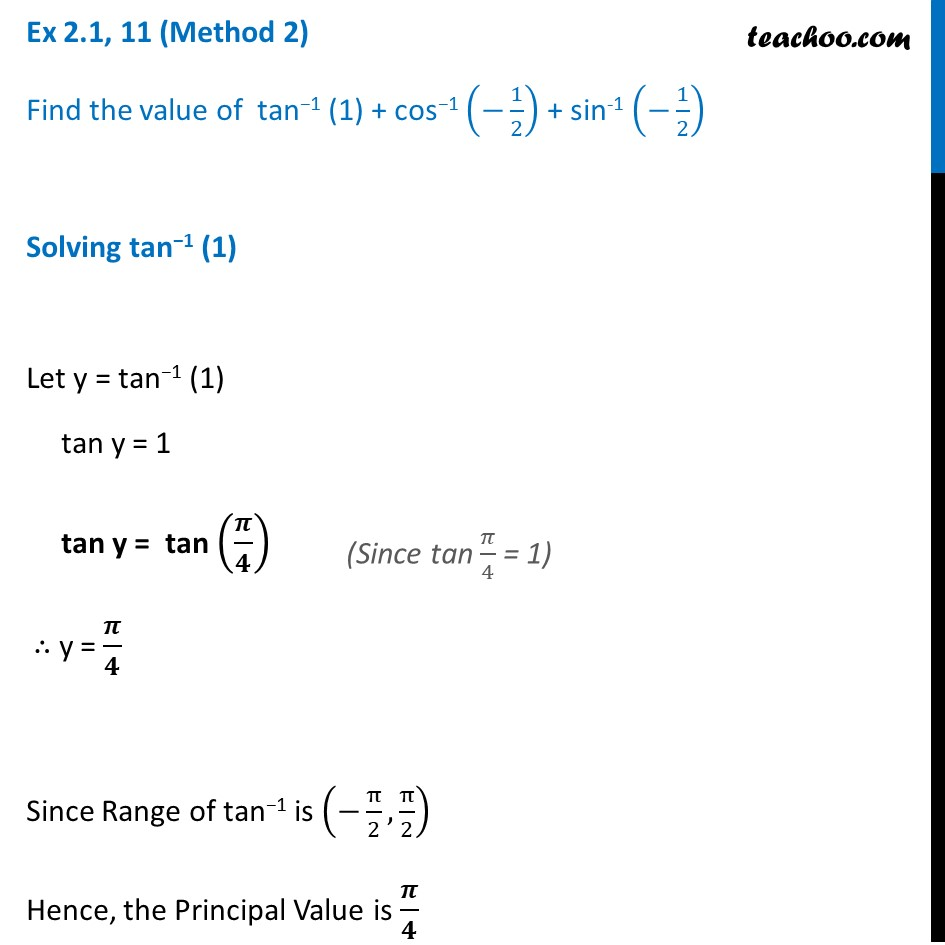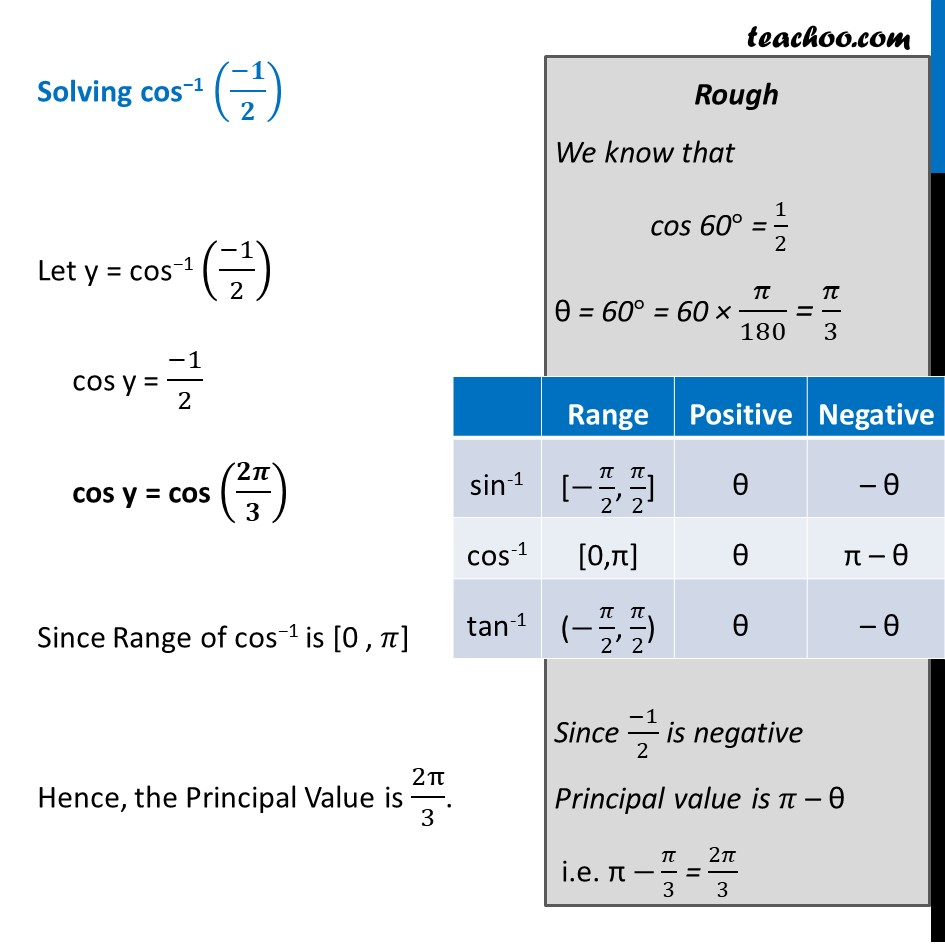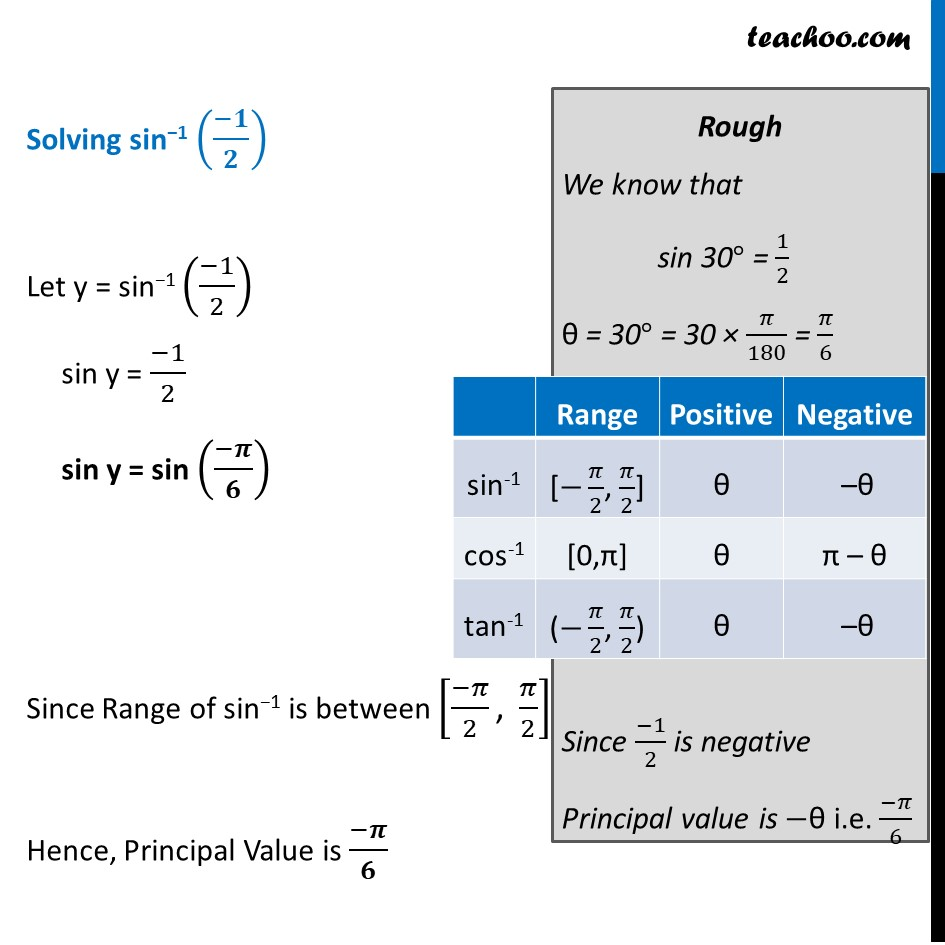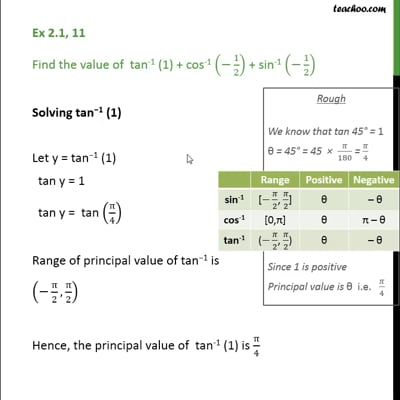This video is only available for Teachoo black users

Solve all your doubts with Teachoo Black (new monthly pack available now!)

### Transcript

Ex 2.1, 11 (Method 1) Find the value of tan−1 (1) + cos−1 (−1/2) + sin-1 (−1/2) Solving tan−1 (1) Let y = tan−1 (1) tan y = 1 tan y = tan (𝝅/𝟒) ∴ y = 𝝅/𝟒 Since Range of tan−1 is (−π/2,π/2) Hence, the Principal Value is 𝝅/𝟒 Solving cos−1 ((−𝟏)/𝟐) Let y = cos−1 ((−1)/2) y = 𝜋 − cos−1 (1/2) y = 𝜋 − 𝝅/𝟑 y = 𝟐𝝅/𝟑 Since Range of cos−1 is [0 , 𝜋] Hence, the Principal Value is 2π/3 We know that cos−1 (−x) = 𝜋 − cos −1 x Since cos 𝜋/3 = 1/2 𝜋/3 = cos−1 (1/2) Solving sin−1 ((−𝟏)/𝟐) Let y = sin−1 ((−1)/2) y = − sin−1 (1/2) y = (−𝝅)/𝟔 Since Range of sin−1 is between [(−𝜋)/2 ", " 𝜋/2] Hence, Principal Value is (−𝝅)/𝟔 We know that sin−1 (−x) = − sin−1 x Since sin 𝜋/6 = 1/2 𝜋/6 = sin−1 (1/2) Now we have tan−1 (1) = π/4 , cos−1 ((−1)/2) = 2π/3 , sin−1 ((−1)/2) = (−π)/6 Finding tan−1 (1) + cos−1 ((−𝟏)/𝟐) + sin−1 ((−𝟏)/𝟐) = π/4 + 2π/3 – π/6 = (3 × π + 4 × (2π) − 2 (π))/12 = (3π + 8π − 2π)/12 = 9π/12 = 𝟑𝛑/𝟒 Ex 2.1, 11 (Method 2) Find the value of tan−1 (1) + cos−1 (−1/2) + sin-1 (−1/2) Solving tan−1 (1) Let y = tan−1 (1) tan y = 1 tan y = tan (𝝅/𝟒) ∴ y = 𝝅/𝟒 Since Range of tan−1 is (−π/2,π/2) Hence, the Principal Value is 𝝅/𝟒 Solving cos−1 ((−𝟏)/𝟐) Let y = cos−1 ((−1)/2) cos y = (−1)/2 cos y = cos (𝟐𝝅/𝟑) Since Range of cos−1 is [0 , 𝜋] Hence, the Principal Value is 2π/3. Rough We know that cos 60° = 1/2 θ = 60° = 60 × 𝜋/180 = 𝜋/3 Since (−1)/2 is negative Principal value is 𝜋 – θ i.e. π −𝜋/3 = 2𝜋/3 Solving sin−1 ((−𝟏)/𝟐) Let y = sin−1 ((−1)/2) sin y = (−1)/2 sin y = sin ((−𝝅)/𝟔) Since Range of sin−1 is between [(−𝜋)/2 ", " 𝜋/2] Hence, Principal Value is (−𝝅)/𝟔 Rough We know that sin 30° = 1/2 θ = 30° = 30 × 𝜋/180 = 𝜋/6 Since (−1)/2 is negative Principal value is −θ i.e. (−𝜋)/6 Now we have tan−1 (1) = π/4 , cos−1 ((−1)/2) = 2π/3 , sin−1 ((−1)/2) = (−π)/6 Finding tan−1 (1) + cos−1 ((−𝟏)/𝟐) + sin−1 ((−𝟏)/𝟐) = π/4 + 2π/3 – π/6 = (3 × π + 4 × (2π) − 2 (π))/12 = (3π + 8π − 2π)/12 = 9π/12 = 𝟑𝛑/𝟒